# Step 1: Measuring and Ordering

Using a tape measure, walk around your yard and measure the area of your planned lawn (perhaps you might like to prepare a quick sketch of the lawn on a piece of paper, be sure to include paths, gardens, pool etc)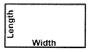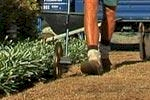Square or Rectangle:

Measure in metres the LENGTH and WIDTH of the Square or Rectangular area. To calculate, multiply the length by the width.

Example: Length 10 metres & Width 5 metres – 10 x 5 = 50 square metres.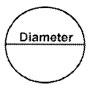Circle:

Measure in metres the DIAMETER of the Circular area. To calculate, multiply by 0.80 by the diameter and then by diameter again.

Example: Diameter 9 metres – 0.80 x 9 x 9 = 64.80 square metres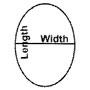Oval:

Measure in metres the DIAMETER of the Circular area. To calculate, multiply by 0.80 by the diameter and then by diameter again.

Example: Diameter 9 metres – 0.80 x 9 x 9 = 64.80 square metres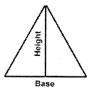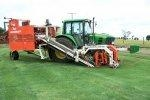Triangle:

Measure in metres the BASE and HEIGHT of the Triangular area. To calculate, multiply 0.50 by the base and then by the height.

Example: Base 40 metres & height 25 metres – 0.50 x 40 x 25 = 500 square metres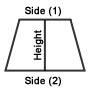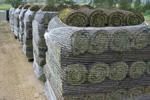Trapezoid:

Measure in metres PARALLEL SIDES (1) and (2) and HEIGHT perpendicular to the Parallel Sides. To calculate, multiply 0.50 by the sum of the two parallel sides and then by the height.

Example: Parallel side (1) 30 metres: parallel side (2) 50 metres: height 20 metres

0.50 x (30+50=80) x 20 = 800 square metres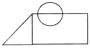Unusual Shapes:Measure metres by firstly dividing area into sections of regular shapes ie: Circle, Square and Triangle. To calculate, apply formulas as mentioned above for each shape.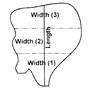Different Widths:

Measure metres by firstly measuring the length of the longest axis across the area. Every two metres along the length line measure the width of the area at right angles to the length line. Total all widths, divide by number of width section and multiply by length.

Example: Length 9 metres: width (1) 3 metres: width (2) 5 metres: width (3) 4 metres.

3+5+4=12 divide by 3 = 4 x 9 = 36 square metres

Step 2: Site and Soil Preparation